# CBSE Class 12 Physics Electric Charges and Fields MCQs

## Electric Charges and Fields Class 12 Physics MCQ

Class 12 Physics students should refer to the following multiple-choice questions with answers for Electric Charges and Fields in standard 12. These MCQ questions with answers for Grade 12 Physics will come in exams and help you to score good marks

### Electric Charges and Fields MCQ Questions Class 12 Physics with Answers

Question: In figure two positive charges q2 and q3 fixed along the y-axis, exert a net electric force in the + x-direction on a charge q1 fixed along the x-axis. If a positive charge Q is added at (x, 0), the force on q1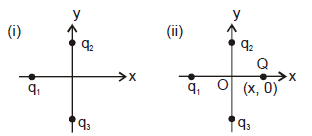(a) shall increase along the positive x-axis

(b) shall decrease along the positive x-axis

(c) shall point along the negative x-axis

(d) shall increase but the direction changes because of the intersection of Q with q2 and q3

Question: A point positive charge is brought near an isolated conducting sphere (figure). The electric field is best given by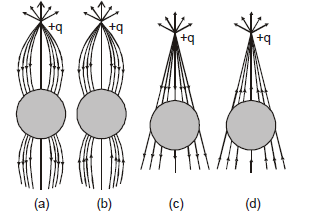Question: The electric flux through the surface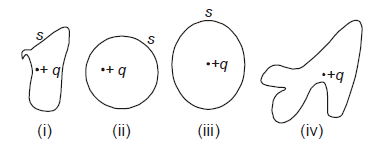(a) in Fig. (iv) is the largest

(b) in Fig. (iii) is the least

(c) in Fig. (ii) is same as Fig. (iii) but is smaller than Fig. (iv)

(d) is the same for all the figures

Question: Figure shows electric field lines in which an electric dipole P is placed as shown. Which of the following statements is correct?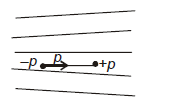(a) The dipole will not experience any force

(b) The dipole will experience a force towards right

(c) The dipole will experience a force towards left

(d) The dipole will experience a force upwards

Question: A point charge + q is placed at a distance d from an isolated conducting plane. The field at a point P on the other side of the plane is

(a) directed perpendicular to the plane and away from the plane

(b) directed perpendicular to the plane but towards the plane

(c) directed radially away from the point charge

(d) directed radially towards the point charge

Question:  A hemisphere is uniformely charged positively. The electric field at a point on a diameter away from the centre is directed

(a) perpendicular to the diameter

(b) parallel to the diameter

(c) at an angle tilted towards the diameter

(d) at an angle tilted away from the diameter

Question: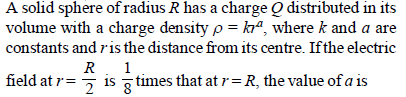(a) 3

(b) 5

(c) 2

(d) 7

Question: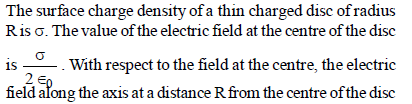(a) reduces by 70.7%

(b) reduces by 29.3%

(c) reduces by 9.7%

(d) reduces by 14.6%

Question: Assertion : The positive charge particle is placed in front of a spherical uncharged conductor. The number of lines of forces terminating on the sphere will be more than those emerging from it.
Reason : The surface charge density at a point on the sphere nearest to the point charge will be negative and maximum in magnitude compared to other points on the sphere.

(a) If both Assertion and Reason are correct and Reason is the correct explanation of Assertion.

(b) If both Assertion and Reason are correct, but Reason is not the correct explanation of Assertion.

(c) If Assertion is correct but Reason is incorrect.

(d) If both the Assertion and Reason are incorrect.

Question: An electron falls from rest through a vertical distance h in a uniform and vertically upward directed electric field E. The direction of electric field is now reversed, keeping its magnitude the same. A proton is allowed to fall from rest in it through the same vertical distance h. The time of fall of the electron, in comparison to the time of fall of the proton is

(a) smaller

(b) 5 times greater

(c) equal

(d) 10 times greater

Question: Charge is the property associated with matter due to which it produces and experiences

(a) electric effects only
(b) magnetic effects only
(c) both electric and magnetic effects
(d) None of these

Question: Charge is
(a) transferable
(b) associated with mass
(c) conserved
(d) All of these

Question: A body is positively charged, it implies that
(a) there is only positive charge in the body.
(b) there is positive as well as negative charge in the body but the positive charge is more than negative charge
(c) there is equal positive and negative charge in the body but the positive charge lies in the outer regions
(d) negative charge is displaced from its position

Question: On rubbing, when one body gets positively charged and other negatively charged, the electrons transferred from positively charged body to negatively charged body are
(a) valence electrons only
(b) electrons of inner shells
(c) both valence electrons and electrons of inner shell
(d) yet to be established

Question: Which of the following is the best insulator?
(a) Carbon
(b) Paper
(c) Graphite
(d) Ebonite

Question: If a body is negatively charged, then it has
(a) excess of electrons (b) excess of protons
(c) deficiency of electrons (d) deficiency of neutrons

Question: When a body is charged by induction, then the body
(a) becomes neutral
(b) does not lose any charge
(c) loses whole of the charge on it
(d) loses part of the charge on it

Question: On charging by conduction, mass of a body may
(a) increase
(b) decreases
(c) increase or decrease
(d) None of these

Question: Quantisation of charge implies
(a) charge cannot be destroyed
(b) charge exists on particles
(c) there is a minimum permissible charge on a particle
(d) charge, which is a fraction of a coulomb is not possible.

Question: If an object possesses an electric charge, it is said to be electrified or ... A ... When it has no charge, it is said to be ... B ... Here, A and B refer to
(a) charged, neutral
(b) neutral, charged
(c) discharged, charged
(d) active, reactive

Question: A positively charged rod is brought near an uncharged conductor. If the rod is then suddenly withdrawn, the charge left on the conductor will be
(a) positive
(b) negative
(c) zero
(d) cannot say

Question: Two spheres A and B of exactly same mass are given equal positive and negative charges respectively. Their masses after charging
(a) remains unaffected
(b) mass of A > mass of B
(c) mass of A < mass of B
(d) Nothing can be said

Question: When a comb rubbed with dry hair attracts pieces of paper. This is because the
(a) comb polarizes the piece of paper
(b) comb induces a net dipole moment opposite to the direction of field
(c) electric field due to the comb is uniform
(d) comb induces a net dipole moment perpendicular to the direction of field

Question: When some charge is transferred to ...A... it readily gets distributed over the entire surface of ... A... If some charge is put on ... B..., it stays at the same place.
Here, A and B refer to
(a) insulator, conductor
(b) conductor, insulator
(c) insulator, insulator
(d) conductor, conductor

Question: Quantisation of charge was experimentally demonstrated by
(a) Einstein’s photoelectric effect
(b) Frank-Hertz experiment
(c) Davisson and Germer experiment
(d) Millikan’s oil drop experiment

Question: In annihilation process, in which an electron and a positron transform into two gamma rays, which property of electric charge is displayed?
(b) Quantisation of charge
(c) Conservation of charge
(d) Attraction and repulsion

Question: The law, governing the force between electric charges is known as
(a) Ampere's law
(b) Ohm's law
(d) Coulomb's law

Question: The value of electric permittivity of free space is
(a) 9 × 109 NC2/m2
(b) 8.85 × 10–12Nm2/C2 sec
(c) 8.85 × 10–12 C2/Nm2
(d) 9 × 109C2/Nm2

Question: Coulomb’s law is true for
(a) atomic distances (= 10–11 m)
(b) nuclear distances (= 10–15 m)
(c) charged as well as uncharged particles
(d) all the distances

Question: What happens when some charge is placed on a soap bubble?
(c) The bubble collapses
(d) None of these

Question: Two point charges + Q and + q are separated by a certain distance. If + Q > + q then in between the charges the electric field is zero at a point
(a) closer to + Q
(b) closer to + q
(c) exactly at the mid-point of line segment joining + Q and + q.
(d) no where on the line segment joining + Q and + q.

Question: An electric field can deflect
(a) neutrons
(b) X-rays
(c) γ-rays
(d) α-particles

Question: The unit of electric field is not equivalent to
(a) N / C
(b) J / C
(c) V / m
(d) J / Cm

Question: If an electron has an initial velocity in a direction different from that of an electric field, the path of the electron is
(a) a straight line
(b) a circle
(c) an ellipse
(d) a parabola

Question: A charged particle is free to move in an electric field. It will travel
(a) always along a line of force
(b) along a line of force, if its initial velocity is zero
(c) along a line of force, if it has some initial velocity in the
direction of an acute angle with the line of force
(d) none of the above

Question: If a linear isotropic dielectric is placed in an electric field of strength E, then the polarization P is
(a) independent of E
(b) inversely proportional to E
(c) directly proportional to E
(d) directly proportional to E

Question: A point charge is kept at the centre of metallic insulated spherical shell. Then
(a) electric field out side the sphere is zero
(b) electric field inside the sphere is zero
(c) net induced charge on the sphere is zero
(d) electric potential inside the sphere is zero

Question: If one penetrates a uniformly charged spherical cloud, electric field strength
(a) decreases directly as the distance from the centre
(b) increases directly as the distance from the centre
(c) remains constant
(d) None of these

Question: Electric lines of force about a negative point charge are
(a) circular anticlockwise
(b) circular clockwise

Question: Electric lines of force
(a) exist everywhere
(b) exist only in the immediate vicinity of electric charges
(c) exist only when both positive and negative charges are near one another
(d) are imaginary

Question: Positive electric flux indicates that electric lines of force are directed
(a) outwards
(b) inwards
(c) either (a) or
(b) (d) None of these

Question: The S.I. unit of electric flux is
(a) weber
(b) newton per coulomb
(c) volt × metre
(d) joule per coulomb

Question: If the flux of the electric field through a closed surface is zero, then
(a) the electric field must be zero everywhere on the surface
(b) the electric field may not be zero everywhere on the surface
(c) the charge inside the surface must be zero
(d) the charge in the vicinity of the surface must be zero

Question: Electric flux over a surface in an electric field may be
(a) positive
(b) negative
(c) zero
(d) All of the above

Question: The electric field inside a spherical shell of uniform surface charge density is
(a) zero
(b) constant different from zero
(c) proportional to the distance from the curve
(d) None of the above

Question: For distance far away from centre of dipole the change in magnitude of electric field with change in distance from the centre of dipole is
(a) zero.
(b) same in equatorial plane as well as axis of dipole.
(c) more in case of equatorial plane of dipole as compared to axis of dipole.
(d) more in case of axis of dipole as compared to equatorial plane

Question: A region surrounding a stationary electric dipoles has
(a) magnetic field only
(b) electric field only
(c) both electric and magnetic fields
(d) no electric and magnetic fields

Question: The electric field at a point on equatorial line of a dipole and direction of the dipole moment
(a) will be parallel
(b) will be in opposite direction
(c) will be perpendicular
(d) are not related

Question: Debye is the unit of
(a) electric flux
(b) electric dipole moment
(c) electric potential
(d) electric field intensity

Question: An electric dipole will experience a net force when it is placed in
(a) a uniform electric field
(b) a non-uniform electric field
(c) both (a) and (b)
(d) None of these

Question: An electric dipole is kept in a non-uniform electric field. It experiences
(a) a force and a torque
(b) a force but not a torque
(c) a torque but no force
(d) neither a force nor a torque

Question: The formation of a dipole is due to two equal and dissimilar point charges placed at a
(a) short distance
(b) long distance
(c) above each other
(d) none of these

Question: A hollow sphere of charge does not have electric field at
(a) outer point
(b) interior point
(c) beyond 2 m
(d) beyond 100 m

Question: Charge motion within the Gaussian surface gives changing physical quantity
(a) electric field
(b) electric flux
(c) charge
(d) gaussian surface area

Question: Gauss’s law is true only if force due to a charge varies as
(a) r–1
(b) r–2
(c) r–3
(d) r–4

Question: An electric dipole is placed at an angle of 30° to a non-uniform electric field. The dipole will experience
(a) a translational force only in the direction of the field
(b) a translational force only in the direction normal to the direction of the field
(c) a torque as well as a translational force
(d) a torque only

Question: An electric dipole is placed at the centre of a sphere then
(a) the flux of the electric field through the sphere is not zero.
(b) the electric field is zero at every point of the sphere.
(c) the electric field is not zero anywhere on the sphere.
(d) the electric field is zero on a circle on the sphere.

Question: Gauss's law is valid for
(a) any closed surface
(b) only regular close surfaces
(c) any open surface
(d) only irregular open surfaces

Question: An electric dipole has a pair of equal and opposite point charges q and –q separated by a distance 2x. The axis of the dipole is
(a) from positive charge to negative charge
(b) from negative charge to positive charge
(c) Perpendicular to the line joining the two charges rawn at the centre and pointing upward direction
(d) Perpendicular to the line joining the two charges drawn at the centre and pointing downward direction

Question: The Gaussian surface
(a) can pass through a continuous charge distribution.
(b) cannot pass through a continuous charge distribution.
(c) can pass through any system of discrete charges.
(d) can pass through a continuous charge distribution as well as any system of discrete charges.

Question:  Assertion : On moving a distance two times the initial distance away from an infinitely long straight uniformly charged wire the electric field reduces to one third of the initial value.

Reason : The electric field is inversely proportional to the distance from an infinitely long straight uniformly charged wire.

(a) Assertion is correct, reason is correct; reason is a correct explanation for assertion.

(b) Assertion is correct, reason is correct; reason is not a correct explanation for assertion

(c) Assertion is correct, reason is incorrect

(d) Assertion is incorrect, reason is correct.

Question:   A charged particle q is placed at the centre O of cube of length L (A B C D E F G H). Another same charge q is placed at a distance L from O. Then the electric flux through ABCD is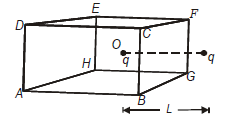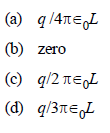Question: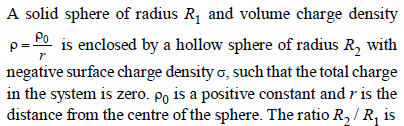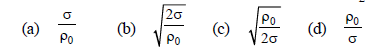Question: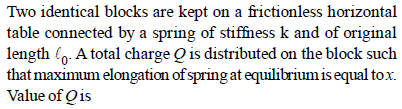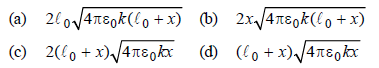Question:  Two small balls having the same mass and charge and located on the same vertical at heights h1 and h2 are thrown in the same direction along the horizontal at the same velocity v. The first ball touches the ground at a distance l from the initial vertical. At what height H2 will the second ball be at this instant ? The air drag and the effect of the charges induced on the ground should be neglected.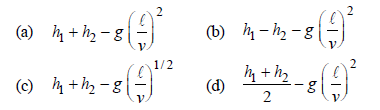Question: 6 A particle of charge q and mass m moves rectilinearly under the action of electric field E = A – Bx, where A and B are positive constants and x is distance from the point where particle was initially at rest then the distance traveled by the particle before coming to rest and acceleration of particle at that moment are respectively :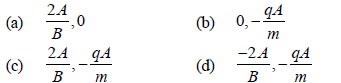Question:  Two identical thin rings, each of radius a meter, are coaxially placed at a distance R meter apart. If Q1 coulomb and Q2 coulomb are respectively the charges uniformly spread on the two rings, the work done in moving a charge q coulomb from the centre of one ring to that of the other is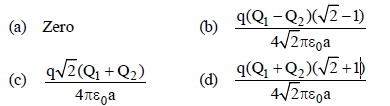Question: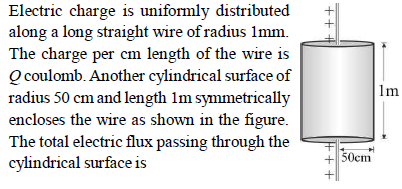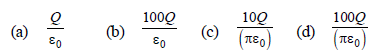Question: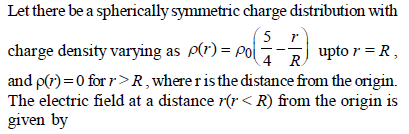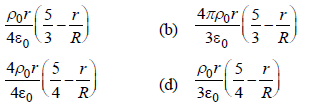Question: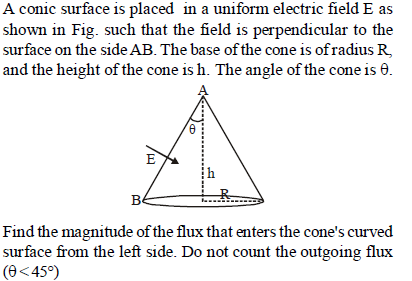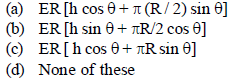CBSE Class 12 Physics Electric Charges and Fields MCQs
 CBSE Class 12 Physics Wave Optics MCQs
 CBSE Class 12 Physics Dual Nature of Radiation and Matter MCQs
 CBSE Class 12 Physics Atoms MCQs
 CBSE Class 12 Physics Nuclei MCQs
 CBSE Class 12 Physics Semiconductor Electronics Materials Devices and Simple Circuits MCQs
 CBSE Class 12 Physics Communication Systems MCQs
 CBSE Class 12 Physics Electrostatic Potential and Capacitance MCQs
 CBSE Class 12 Physics Current Electricity MCQs
 CBSE Class 12 Physics Moving Charges and Magnetism MCQs
 CBSE Class 12 Physics Magnetism and Matter MCQs
 CBSE Class 12 Physics Electromagnetic Induction MCQs
 CBSE Class 12 Physics Alternating Current MCQs
 CBSE Class 12 Physics Electromagnetic Waves MCQs CBSE Class 12 Physics Gravitation MCQs
 CBSE Class 12 Physics Ray Optics and Optical Instruments MCQs